# COVID-19 SIR Modelling for Andhra Pradesh, India

June 13, 2020

By  Manoj sai

###### Move quickly from one page to another within COVID-19 Series from MATLAB Helper:

Now coming on to the various approaches to evaluating the SIR model. Here we will come up with the different mathematical models that predict such as how bad the pandemic can result

In this approach, we are showing the pandemic analysis of one of the states of India that is Andhra Pradesh (AP).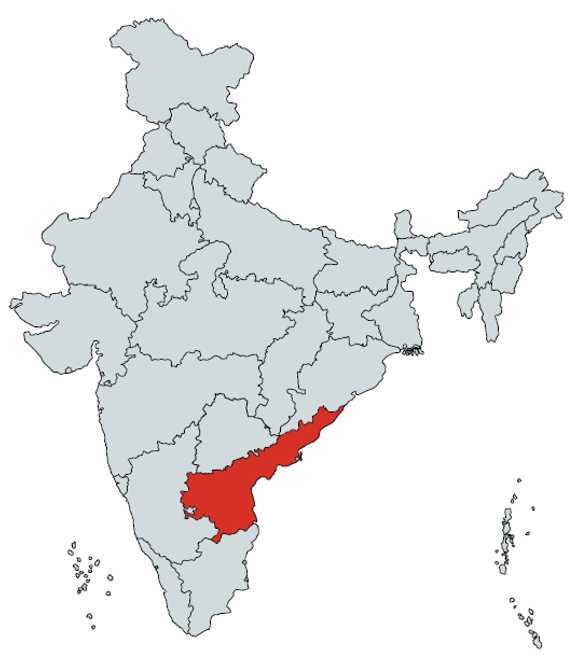Here we are going to take our first assumption, which presumes the only 50,000 of the total population of AP has chances of getting exposed to the virus. Then we are further dividing this population into 3 different categories. So we go on describing them as:

#### Susceptible, S(t)

They are all the people that are capable of becoming sick from an infection caused by the Novel Corona-virus. So, initially, the total exposed population will come under susceptible.

#### Infected, I(t)

When a certain amount of population becomes infected, that proportion leaves the susceptible category. Once many people get infected, then those people are not susceptible to get it a second time. You just get infected once, and that is the assumption as well!

The ones who are now having the disease are going to transition at some point, hopefully into a recovered status.

#### Recovered R(t)

The number of the total population out of the infected category who does not have the disease anymore constitutes the recovered category.

We have also considered one more assumption that the death rate and birth rate are low compared to the number that is being susceptible or recovered and hence therefore negligible to not be considered in these calculations.

We will now imagine that this explains to everyone that either the person is susceptible, which means they could get out of the disease and recover, or they stay infected. If a person is infected, s/he can go and infect other people, or they can even go and be among the recovered person later.

In the recovered case, they can neither get this particular illness, nor they can transmit to somebody else again!

These are assumptions of this model. We can write this assumption as an equation by saying:

N = S(t) + I(t) + R(t)

The sum of S(t), I(t), and R(t) adds up to N, which is perhaps everybody. N is the exposed population.

For evaluating the model, we are now going to give labels to analyse as to what phenomenon is happening to the various values at different intervals of time.

So first let's take

### Case I: T = 0

This is the very beginning of the so-called initial conditions. The idea here is that at the very beginning, there are no recovered people because we are at the beginning where the count for the infected people will also be less in the beginning.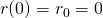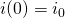i.e. first confirmed case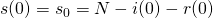Here, what we are trying to do is to write down a set of equations that govern the behavior of S(t), I(t) and R(t). So what we are going to do is to use a type of mathematics called the system of differential equations to let us now know more about SIR deeply. The idea is to compute three parameters for the rate of change with respect to time:

•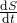••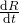For each of these equations, we will write down the rate of change w.r.t time

• dS/dt explains how to number of susceptible is changing with time
• dI/dt explains how to number of Infected people  is changing with time
• dR/dt explains how to number of recovered is changing with time.

The differential equations for the above parameters are:

••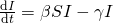•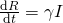Take the first susceptible equation

We can write,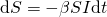This means that for a small change in time we can predict the small change in susceptible population. We can split the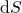as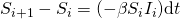Now we can predict the future susceptible value using today's susceptible and infected values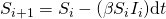In the similar approach we can get for infected and recovered equations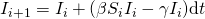Moving to the mathematical model

### Section: Data Fetching

In this section, we will learn to know how to extract data from the URL as an input to our code .

%Getting raw data from the website
url='http://api.covid19india.org/csv/latest/state_wise_daily.csv';

We have taken raw data of the Coronavirus stats from http://api.covid19india.org/csv/latest/state_wise_daily.csv. The data from this URL has the stats for all the Indian states dated from the 14th of March 2020 which has the column-wise stats on 'confirmed,' 'recovered,' and 'deceased.' Pertaining to all the dates, here we go on to a particular state, namely Andhra Pradesh, for a better understanding of the model.

We have saved our data in the variable called 'raw.' The 'raw=webread(url)' command reads content from the web service specified by URL and returns the content in 'url.' The function of webread is to read content from the RESTful web service. The web service provides a RESTful that returns data formatted as an internet media type such as JSON, XML, image, or text.

### Section: Defining Counters and assignment of array

This is the section where we define the counters, namely 'i', 'j' and 'k' and the counters for different arrays.

%Creating confirmed and recovered arrays which will contain the infected
%and recovered cumulative data respectively
j=1;
%Initialising the AP column with the first row containing initial confirmed
%case
i=1;
%Initialising the AP column with second-row containing initial recovered
%case
k=2;
confirmed (j) =0;
recovered (j) =0;
while (i<length(raw.AP))
confirmed (j+1) =confirmed(j)+raw.AP(i);
recovered (j+1) =recovered(j)+raw.AP(k);
j=j+1;
i=i+3;
k=k+3;
end

Now moving on to the variables we have used, namely i,  j and k.  The purpose of the variable j is for the creation of an array of confirmed and recovered stats that will contain the infected and recovered cumulative data, respectively. Our purpose behind taking the data cumulative is because the data provided to us is on daily analysis, so we need to add it to the previous data. While this is cumulative, there is an addition of an extra row, so we don't include the 1st row, which is signified by the command ( i<=length(raw.AP)-1).

Now moving on to the variable 'i,' which is used for initializing the column with the first row containing initial confirmed case for the state AP. Moving further to the variable 'k,' which is used for initializing the column with second-row containing the first recovered case for the state AP.

The number of people who are exposed to the infection has been defined previously to be 50,000 which stored in the variable 'N' and according to our second assumption the number of confirmed and recovered cases to be null which are defined as

confirmed (j) =0;

recovered (j) =0;

The commands i=i+3 and k=k+3 signifies that every  3rd row from the starting initial point is being taken, for, e.g., the 'i' signifies the confirmed cases so in our data model to reach the 3rd row of the confirmed with a new date and the same applies for the variable j.

### Section: Finding the values of our constants

In this section, we will be finding the value of 'beta' and 'gamma' through some pre-defined formulas.

%% Finding constants beta and Gamma from the data

%N is the assumed exposed population in Andhra Pradesh
N=50000;
%"d" is the unique dates frm the raw data
d=unique(raw.Date);
%Creating infected and recovered arrays from "confirmed" and "recovered"
%arrays respectively
infected=confirmed(2:end)' ;
recovered=recovered(2:end)';
%Since N=suspectable+infected+recovered
susceptible=N-infected-recovered;
%The step size is one day
dt=1;

for i=1:(length(susceptible)-1)
b(i)=(susceptible(i)-susceptible(i+1))/(susceptible(i).*infected(i)*dt);
end

for i=1:(length(susceptible)-1)
g(i) =(recovered(i+1)-recovered(i))/( infected(i)*dt ) ;
end

%Take the final value of b and g arrays as beta and gamma respectively
gamma=g(end);
beta=b(end);

Now we move on to find the constants for our model, namely 'beta' and 'gamma.'  Beta is the infection rate of the virus, and the Gamma is the recovery rate.

For taking out these values, we have variable d, which is used for the different dates from the data of the state AP. For this, we have used the function called 'unique.' The function of the unique is to find the unique elements of the vector.

For, eg. If we take b = unique(A), It returns the same values as in A but with no repetitions. The resulting vector is sorted in ascending order. A can be a cell array of strings.

After finding the cumulative data, we should truncate the first element that we initialized as zero.

infected=confirmed(2:end)' ;

recovered=recovered(2:end)';

Then we come on to the mathematics of finding the population being susceptible among the exposed once that is the population of 50,000, so the number of susceptible is given by the equation for which we are taking the interval to be one day.

susceptible=N-infected-recovered;

The data about the susceptible will help us in finding the 'beta' and 'gamma' values to which we will find out from the described formulas. Here we will make use of the 'for loop'. We start by obtaining the value of 'beta' which is denoted by the variable 'b' and is formulated as

b(i) =(susceptible(i)-susceptible(i+1))/(susceptible(i).*infected(i)*dt ) ;

It describes that the value of beta is the ratio of the difference of the susceptible of the two consecutive days and the product of the susceptible and product of infected and the time interval.

The same applies to the 'gamma' which is denoted by the variable 'g' and is formulated as

g(i) =(recovered(i+1)-recovered(i))/( infected(i)*dt ) ;

Then we will store the last values of b(i) and g(i) into variables created, which is denoted by the name 'beta' and 'gamma' respectively. Given,

gamma=g(end);

beta=b(end);

Our purpose behind taking the end values is to make the plots more realistic and practical according to the real situation. Taking the end value gives a gradual change in the plot instead of a sudden spike.

Now we found the constants Beta and Gamma using the actual data till today. Next, we should predict the Susceptible, Infected, and Recovered cases using the SIR equations.

### Section: Initializing values

In this section, we will be finding the value of 'beta' and 'gamma' through some pre-defined formulas.

%%
%Using the calculated "beta" and "gamma" we will generate future
%Susceptible, Infected and Recovered using SIR Model

%Initialising Sucseptable, Infected, and Recovered arrays with last date
%values in the raw data respectively
susc=[susceptible(end) inf];
infec=[infected(end) inf];
rec=[recovered(end) inf];
%The first date of prediction will be equal to last date of actual data
d1=d(end);
%Specify the last date of prediction
d2=datetime(0021,12,30);
d_interval=d1:d2;

%Finding Susceptible, Infected, and Recovered array elements starting from
%today to the specified date in "d2" variable using SIR Model
for i=1:length(d_interval)-1

susc(i+1)=(susc(i)-((beta)*susc(i).*infec(i)*dt));
infec(i+1)=(infec(i) +(((beta)*susc(i).*infec(i))-...
((gamma).*infec(i))) *dt);
rec(i+1) =(rec(i) +(((gamma).*infec(i))*dt));

end
%Rounding the values
calculated_susceptible=round(susc');
calculated_infected=round(infec');
calculated_recovered=round(rec');

Now we need to initialize Susceptible, Infected, and Recovered arrays with last date values in the raw data respectively for this we will be using the commands like

susc=[susceptible(end) inf];

infec=[infected(end) inf];

rec=[recovered(end) inf];

The statement 'susc=[susceptible(end) inf];' can be explained by taking an example as considering Current date to be = x and, yesterday's date to be = y. From the data available, we can predict the analysis for other days too. This command indicates the data for date 'y' using the 'beta' and 'gamma' values. So for the latest analysis on date 'x,' we will have the reports of 'y,' and accordingly are the model will give the output for the 'x' date.

For this pandemic, we have assumed the SIR analysis start date to be the 12th of May 2020 and the last date to the 30th of December 2021, so in this way, our model will present the data for this interval. Please note that this is a Mathematical Model and the real figures can be different based on spread.

Using the SIR equation what we derived above we will find susc, infec, and rec.

After we are done with the task of finding the values of 'Susceptible,' 'Infected,' and 'Recovered' array elements starting from today to the specified date in "d2" variable using SIR Model we need to round them off to the nearest integers as the population can't be expressed as a fraction! For rounding off the values, we have used the function 'round()'.

### Section: Plotting Graphs

In this section, we will be plotting the graphs for our SIR model and hence obtain the results from our calculations.

%% Plot
%Plotting actual data
figure(1);
hold on;
plot(d,susceptible,'b');
plot(d,infected,'r');
plot(d,recovered,'g');
ylim([-2000 52000]);
title('Actual data');
ylabel('exposed population');
legend('Susceptible','Infected','Recovered');
grid on;

By our model, we have obtained two plots. The 1st one is for plotting the actual data, and the 2nd one is for plotting the predicted data along with the actual data.

For Figure 1, i.e. Plot 1, We have a single plot between time interval signified by 'd' on the x-axis, and the various parameters like 'susceptible,' 'infected' and 'recovered' related to the exposed population on the y-axis since are graph was a limiting one with the grid on it, so we had provided a limit to the y-axis too to show the boundaries clearly.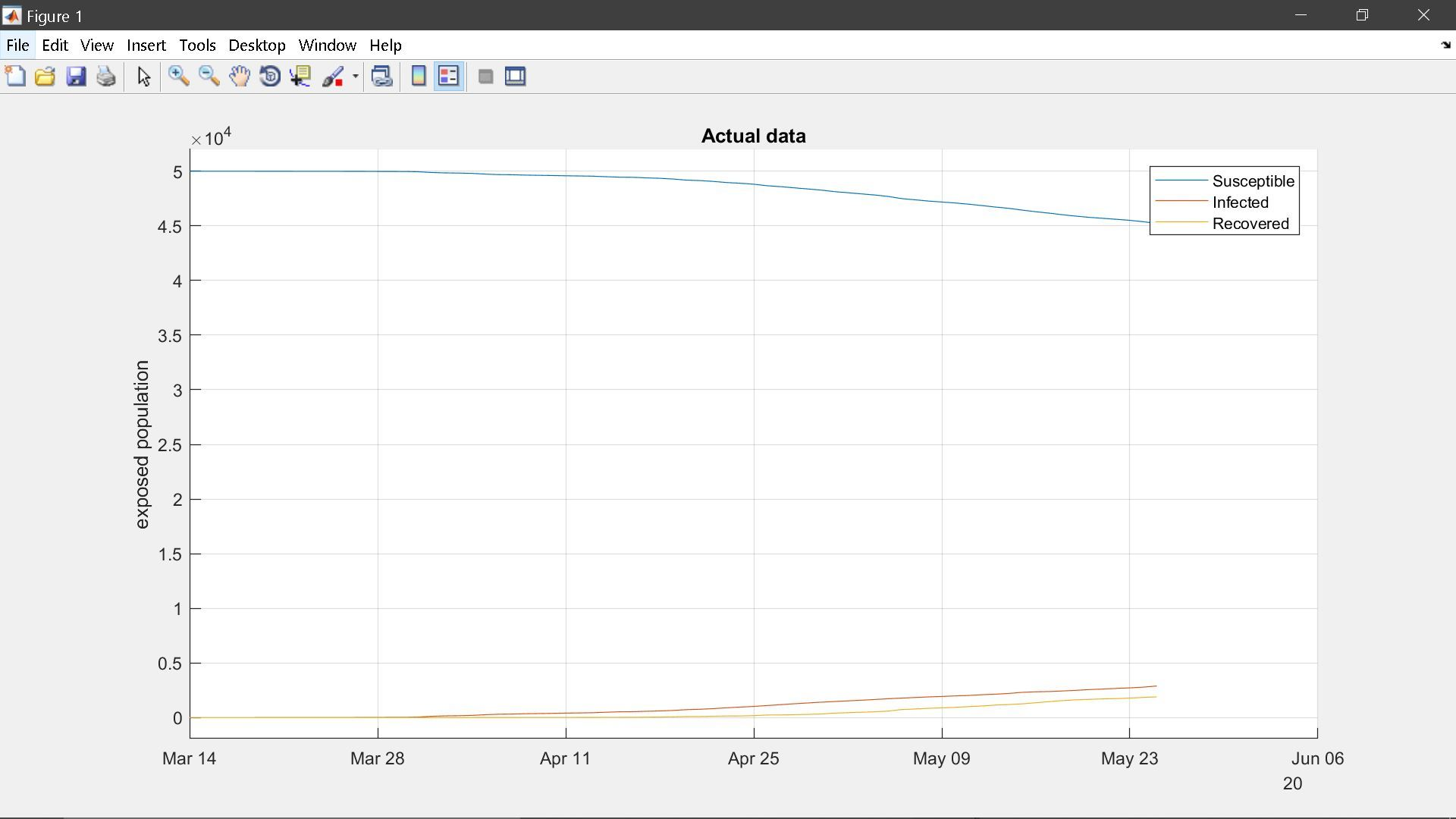%Plotting the predicted data along with the actual data
figure(2);
hold on;
plot(d(:),susceptible,'b',d_interval,calculated_susceptible,'--b');
plot(d(:),infected,'r',d_interval,calculated_infected,'--r');
plot(d(:),recovered,'g',d_interval,calculated_recovered,'--g');
ylim([-2000 52000]);
title('Predicted Data');
ylabel('Exposed Population');
legend('Actual Suceptible','Predicted Future Susceptible',...
'Actual Infected','Predicted Future Infected','Actual Recovered',...
'Predicted Future Recovered');
grid on;

For Figure 2, i.e. the Plot 2, We again have a single plot for the variables, which are the predicted as well as the actual ones we have shown them by broken and continuous lines, respectively, for making the graph to be read easily.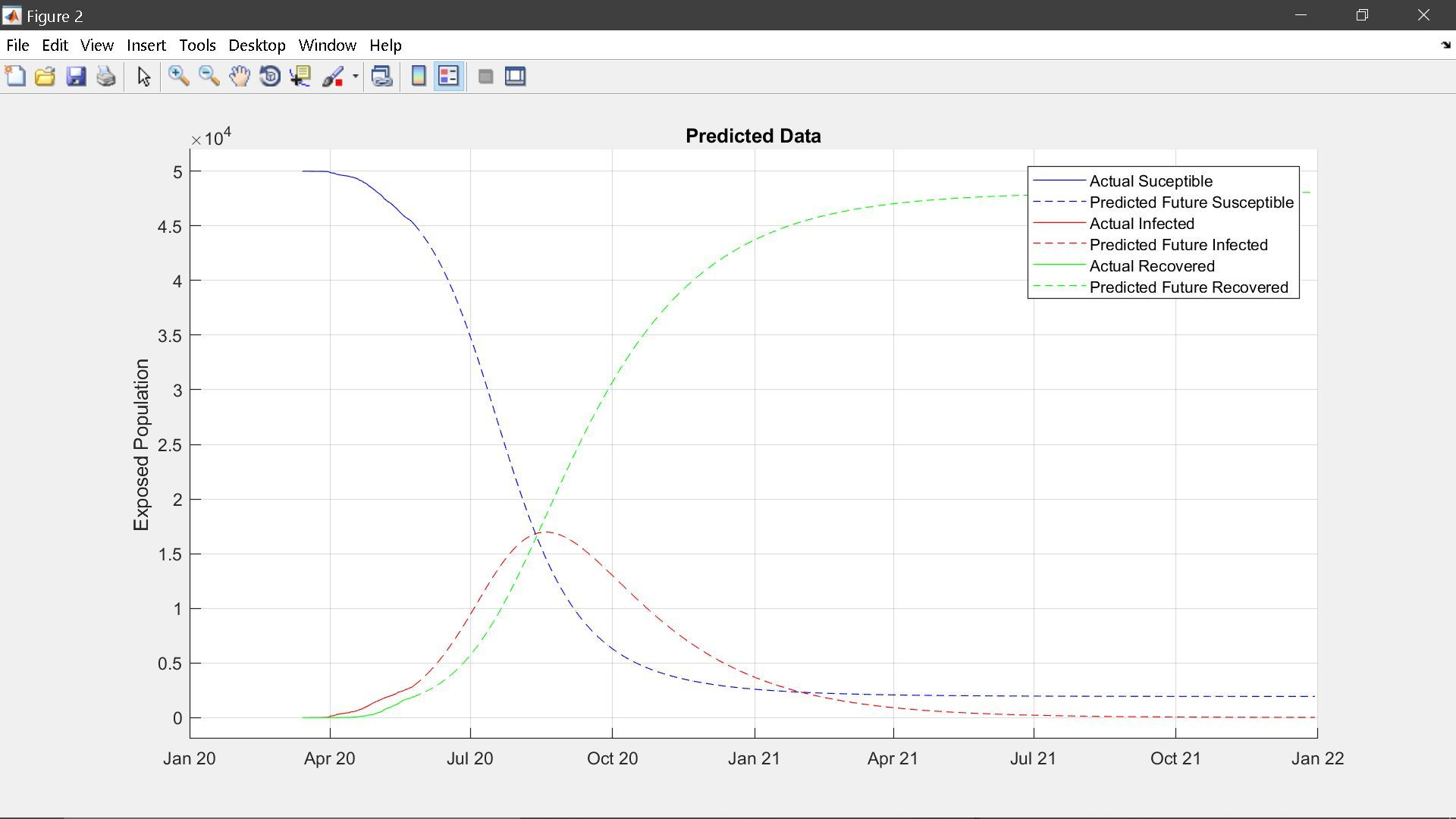Conclusion:

We have used data untill 31st May 2020 for all these analysis. We have seen some approaches to modelling diseases such as COVID-19 through MATLAB. We have found essential characteristics within this process. We have done Data Analysis and Data Extraction and included a reliable source for the COVID-19 data. We have used preprocessing to extract a subset of the collected data and organise the data accurately. We have done Data Modelling with model construction, i.e. finding the best model that fits the COVID-19 pandemic. We have done the calculation of model parameters using real collected data and finally done the Data Visualisation. All these steps were essential, and luckily, MATLAB has so many functions and tool to use in the given scenario.

Now summing our analysis and coming to a definite conclusion, India's COVID-19 case tally climbed from 100 to one lakh in just 64 days as per the data sourced from the Union Ministry of Health & Family Welfare (MoHFW). On comparing this with global data, sourced from Worldometers, it has been found out that India's COVID-19 growth rate is more than double the number of days as compared to Colombia.

### Important Note

Our COVID-19 codes and analysis have been made for educational and research purpose. We have shown different approaches of Pandemic Modelling for each state and the accuracy of result is not guaranteed for real-life situation. We wish for early end of this pandemic. Now it's in our hands; we need to take our responsibilities & take proper precautions.

Now let's have a quick view of the precautions.

#### Stay Home for non-essential activities!Avoid going to crowded areas. Work From Home and Be A Super Hero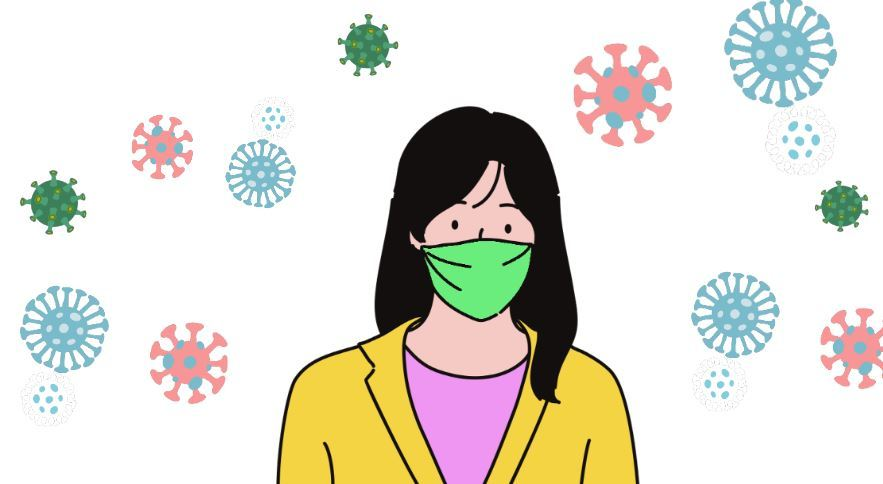Maintain distance while conversing with someone. Avoid touching your face frequently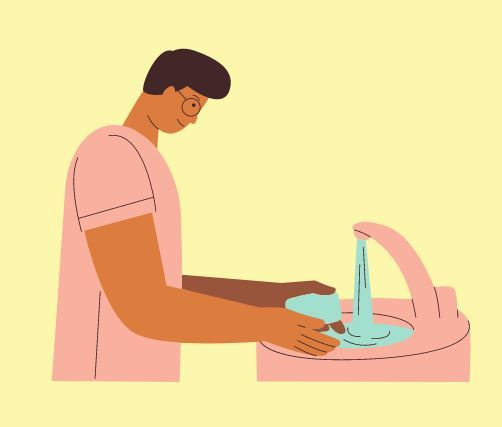Wash your hand for 20 seconds.  KEEP A SANTISER ALWAYS with you, wherever you go.While sneezing or coughing, always cover your mouth with a handkerchief or with your arm.

#### Home Quarantine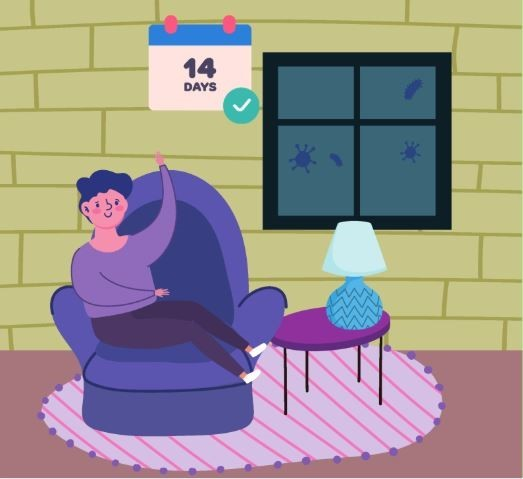If you have been facing the symptoms of Cold and Cough or the one's shown with COVID-19, then self-isolate yourself with home quarantine for 14 days.  So stay home stay safe; this is the best way you can contribute to the betterment of the nation.

The Blog has been created by Manoj Sai along with contributions or modifications from Nikita Mahoviya.

#### Manoj sai

I am currently pursuing b.tech, always interested to learn new things, and a good listener. I strongly believe that " Dream, Dream Dream, Dreams transform into thoughts and thoughts result in action." - Abdul Kalam

•Nikita Mahoviya says:

The code and its explanation is commendable; even a beginner can understand what each line means

•K Manoj sai says:

Thank you????

#### MATLAB Helper ®

Use Website Chat or WhatsApp at +91-8104622179